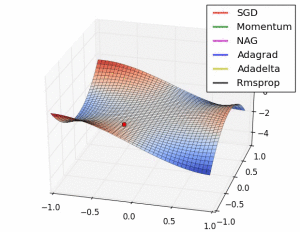← back to “Introduction to Neural Machine Translation with GPUs (Part 2)”

# Figure 10. Behaviour of adaptive learning rate algorithms at a saddle point. From http://imgur.com/a/HqolpFigure 10. Behaviour of adaptive learning rate algorithms at a saddle point. From http://imgur.com/a/Hqolp

Figure 10. Behaviour of adaptive learning rate algorithms at a saddle point. From http://imgur.com/a/Hqolp